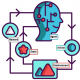###### Understanding Metaclasses in Python
2019-12-04###### Understand Q-Learning in Reinforcement Learning with a numerical example and Python implementation
2019-12-06## Introduction

One of my favorite algorithms that I learned while taking a reinforcement learning course was q-learning. Probably because it was the easiest for me to understand and code, but also because it seemed to make sense. In this quick post, I’ll discuss q-learning and provide the basic background to understanding the algorithm.

## What is q-learning?

Q-learning is an off-policy reinforcement learning algorithm that seeks to find the best action to take given the current state. It’s considered off-policy because the q-learning function learns from actions that are outside the current policy, like taking random actions, and therefore a policy isn’t needed. More specifically, q-learning seeks to learn a policy that maximizes the total reward.

## What’s ‘Q’?

The ‘q’ in q-learning stands for quality. Quality in this case represents how useful a given action is in gaining some future reward.

## Create a q-table

When q-learning is performed we create what’s called a q-table or matrix that follows the shape of `[state, action]` and we initialize our values to zero. We then update and store our q-values after an episode. This q-table becomes a reference table for our agent to select the best action based on the q-value.

`import numpy as np# Initialize q-table values to 0Q = np.zeros((state_size, action_size))`

The next step is simply for the agent to interact with the environment and make updates to the state action pairs in our q-table `Q[state, action]`.

Taking Action: Explore or Exploit

An agent interacts with the environment in 1 of 2 ways. The first is to use the q-table as a reference and view all possible actions for a given state. The agent then selects the action based on the max value of those actions. This is known as exploiting since we use the information we have available to us to make a decision.

The second way to take action is to act randomly. This is called exploring. Instead of selecting actions based on the max future reward we select an action at random. Acting randomly is important because it allows the agent to explore and discover new states that otherwise may not be selected during the exploitation process. You can balance exploration/exploitation using epsilon (ε) and setting the value of how often you want to explore vs exploit. Here’s some rough code that will depend on how the state and action space are setup.

`import random# Set the percent you want to exploreepsilon = 0.2if random.uniform(0, 1) < epsilon:    """    Explore: select a random action    """else:    """    Exploit: select the action with max value (future reward)    """`

Updating the q-table

The updates occur after each step or action and end when an episode is done. Done in this case means reaching some terminal point by the agent. A terminal state for example can be anything like landing on a checkout page, reaching the end of some game, completing some desired objective, etc. The agent will not learn much after a single episode, but eventually, with enough exploring (steps and episodes) it will converge and learn the optimal q-values or q-star (`Q∗`).

Here are the 3 basic steps:

1. Agent starts in a state (s1) takes an action (a1) and receives a reward (r1)
2. Agent selects action by referencing Q-table with the highest value (max) OR by random (epsilon, ε)
3. Update q-values

Here is the basic update rule for q-learning:

`# Update q valuesQ[state, action] = Q[state, action] + lr * (reward + gamma * np.max(Q[new_state, :]) — Q[state, action])`

In the update above there are a couple of variables that we haven’t mentioned yet. What’s happening here is we adjust our q-values based on the difference between the discounted new values and the old values. We discount the new values using the gamma and we adjust our step size using the learning rate (lr). Below are some references.

Learning Rate: `lr` or learning rate, often referred to as alpha orα, can simply be defined as how much you accept the new value vs the old value. Above we are taking the difference between new and old and then multiplying that value by the learning rate. This value then gets added to our previous q-value which essentially moves it in the direction of our latest update.

Gamma: `gamma` or γ is a discount factor. It’s used to balance immediate and future rewards. From our update rule above you can see that we apply the discount to the future reward. Typically this value can range anywhere from 0.8 to 0.99.

Reward: `reward` is the value received after completing a certain action at a given state. A reward can happen at any given time step or only at the terminal time step.

Max: `np.max()` uses the numpy library and is taking the maximum of the future reward and applying it to the reward for the current state. What this does is impact the current action by the possible future reward. This is the beauty of q-learning. We’re allocating future rewards to current actions to help the agent select the highest return action at any given state.

Conclusion

Well, that’s it, short and sweet (hopefully). We discussed that q-learning is an off-policy reinforcement learning algorithm. We show the basic update rule for q-learning using some basic python syntax and we reviewed the required inputs to the algorithm. We learned that q-learning uses future rewards to influence the current action given a state and therefore helps the agent select the best actions that maximize total reward.

There is a lot more on q-learning but hopefully, this is enough to get you started and interested in learning more. I added several resources below that I found helpful when learning about q-learning. Enjoy!

Resources

1. Great RL and q-learning example using the OpenAI Gym taxi environment
2. Reinforcement Learning: An Introduction (free book by Sutton)
3. Quora Q-learning
4. Wikipedia Q-learning
5. David Silver’s lectures on RL

http://kvfrans.com/simple-algoritms-for-solving-cartpole/

https://www.learndatasci.com/tutorials/reinforcement-q-learning-scratch-python-openai-gym/

https://medium.com/@m.alzantot/deep-reinforcement-learning-demystified-episode-0-2198c05a6124

https://towardsdatascience.com/reinforcement-learning-101-e24b50e1d292

https://www.freecodecamp.org/news/a-brief-introduction-to-reinforcement-learning-7799af5840db/

https://medium.com/@m.alzantot/deep-reinforcement-learning-demysitifed-episode-2-policy-iteration-value-iteration-and-q-978f9e89ddaa

https://medium.com/@curiousily/solving-an-mdp-with-q-learning-from-scratch-deep-reinforcement-learning-for-hackers-part-1-45d1d360c120

https://www.freecodecamp.org/news/an-introduction-to-q-learning-reinforcement-learning-14ac0b4493cc/

https://blog.floydhub.com/an-introduction-to-q-learning-reinforcement-learning/

http://mnemstudio.org/path-finding-q-learning-tutorial.htm

Simple Beginner’s guide to Reinforcement Learning & its implementation##### Amir Masoud Sefidian
Machine Learning Engineer